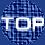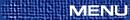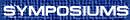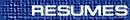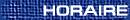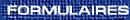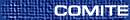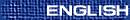Universal Algebra / Algèbre universelle
(J. Hyndman and S. Wismath, Organizers)

JI YOUNG CHOI, Iowa State
The dimension of a finite algebra

Let A be a finite algebra, of size n. Let V be the variety generated by A. Then the dimension of A is the smallest power d of A such that Ad contains a subalgebra that is free in the variety V. Note that d is well-defined, since it is at most equal to n. The concept originates in the theory of permutation groups, where it is also known as the ``minimal base size''. We discuss the problem of determining the dimension of a given finite algebra.

G. GRATZER, Department of Mathematics, University of Manitoba, Winnipeg, Manitoba  R3T 2N2
The case of the shrinking proof: The Dilworth Theorem on embedding every lattice into a uniquely complemented lattice

Let L be a lattice, and let a < b < c be elements of L. We adjoin freely a relative complement u of b in [a,c] to L to form the lattice K. For two polynomials A and B over L È{u}, we find a very simple set of conditions under which A and B represent the same element in K. It follows readily that in K all pairs of relative complements in [a, c] can be described.

As an immediate consequence, we get the classical result of R. P. Dilworth: Every lattice can be embedded into a uniquely complemented lattice. We also get the stronger form due to C. C. Chen and G. Grätzer: Every at most uniquely complemented bounded lattice has a {0,1}-embedding into a uniquely complemented lattice. Some stronger forms of these result are also presented.

The proof by Chen and Grätzer cut roughly in half the proof of Dilworth; the present approach cuts roughly in half the proof of Chen and Grätzer. Maybe, someday, there will be a really short proof that can be presented as a footnote?

B. LAROSE, Concordia University and Champlain Regional College
Constraint satisfaction problems, posets and Taylor operations

Let T be a finite relational structure. Consider the following decision problem: given a structure S of the same type as T, is there a homomorphism from S to T? It has been conjectured that every such problem is either tractable or NP-complete, and that the dichotomy is related to the existence of certain idempotent operations preserving the basic relations of the target structure. We present a short survey of recent results in this area, as well as some related results on retraction and extendibility problems for finite posets. (Joint work with A. Krokhin)

JB NATION, University of Hawaii, Honolulu, Hawaii  96822, USA
A gluing construction for projective planes

A projective plane is a simple, complemented, modular lattice of height 3. We describe a gluing construction which can sometimes be used to make a plane of order n from planes of smaller order. A key step in the construction involves finding directed graphs which are a generalization of symmetric (v,k,l) block designs. These graphs are interesting in their own right.

B. SANDS, University of Calgary, Calgary, Alberta  T2N 1N4
Finite distributive lattices and the splitting property

Given a maximal antichain A of an ordered set P, say that A splits if there is a partition A=BÈC such that P=B­ ÈC¯, where

 B­={x Î P:x ³ b for some b Î B}   and    C¯ = {x Î P:x £ c for some c Î C}.
Say that P has the splitting property if every maximal antichain of P splits. Ahlswede, Erdös and Graham introduced these notions in 1995 and proved that every finite Boolean lattice has the splitting property.

We characterize all finite distributive lattices with the splitting property. It turns out that there are exactly three others besides Boolean lattices.

We also introduce the notion of splitting number, which measures how close a finite ordered set is to being splitting, give what we know of this concept, and state some interesting problems. We then concentrate on specific classes of distributive lattices, so-called ``grids'' obtained as the product of two chains.

M. VALERIOTE, McMaster University, Hamilton, Ontario  L8S 4K1
Varieties with few finite members

The G-spectrum of a variety V is the function gV: N® N, where gV(n) is equal to the number of n-generated members of V, up to isomorphism. The study of G-spectra was initiated by Berman and Idziak in the 1990s.

In my talk I will discuss a result with Idziak and McKenzie on finitely generated varieties whose G-spectra are polynomially bounded. It turns out that these varieties have very nice structure.

R. WILLARD, University of Waterloo, Waterloo, Ontario  N2L 3G1
Failure of injectivity in finitely generated quasivarieties

In 1997 I introduced an ordinal-valued function measuring the degree to which a finite algebra fails to be injective in the quasivariety it generates. (The motivation for such a function comes from the ``natural duality theory'' of D. Clark and B. Davey.) In this talk I will describe the progress that J. Hyndman and I are making in our attempt to understand this function within the context of unary algebras.